# How to change column number with letter in Microsoft Excel

To change in Excel column number to a letter, we need to use the "IF", "AND", "CHAR", "INT" and "MOD" functions in Microsoft Excel 2010.

Before converting the column number to a letter,let us evaluate each formula.

IF: - Checks whether a condition is met and returns one value if True and another value if False.

Syntax of “IF” function =if(logical test,[value_if_true],[value_if_false])

First the formula will perform the logical test.  It will return one value if the output of the logical test is true, else it will return false.

For example:Cells A2 and A3 contain the numbers 3 and 5.  If the number is 3, the formula should display “Yes” otherwise “No”.

=IF (A1=3,"Yes","No")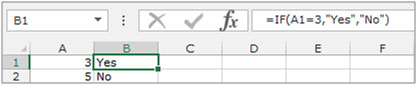AND:- This function is used to check whether all arguments are true and returns TRUE if all arguments are TRUE.  Even if one argument is false, it returns false.

The syntax of “AND” function =AND(logical1,[logical2],….)

For example:Cell A1 contains student name, B1 contains 50, and we need to check if the number is more than 40 and less than 60.

• Write the formula in cell C1
• =AND(B2>40,B2<60), press Enter on the keyboard.
• The function will return True.
• If wechange the criteria to less than 40 and greater than 60, the function will return False.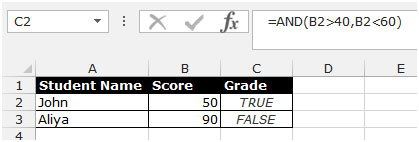INT: - This function is used to round a number down to the nearest integer.

Syntax of “INT” function:  =INT (number)

Example:Cell A1 contains the number 456.25

=INT (A1), function will return 456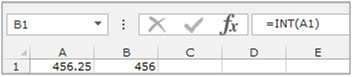MOD: - This function is used to return the remainder after a number is divided by a divisor.

Syntax of “MOD” function:            =MOD (number, divisor)

Example:Cell A1 contains the number 5, and cell A2 contains 7

=MOD (A1,A2), function will return 5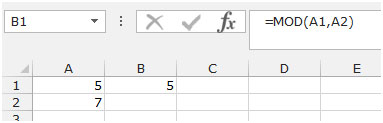The IF function will check the logical test, the AND function will check multiple criteria, the CHAR function will provide the character as per the number, the INT function will round the number down and the Mod function will return the balance for completing the calculation in Microsoft Excel.

Let’s take an example to understand how we can change the column number to a letterin Microsoft Excel.

We have a serial no in column A. In column B, we need to put a formula to convert the numbers to letters.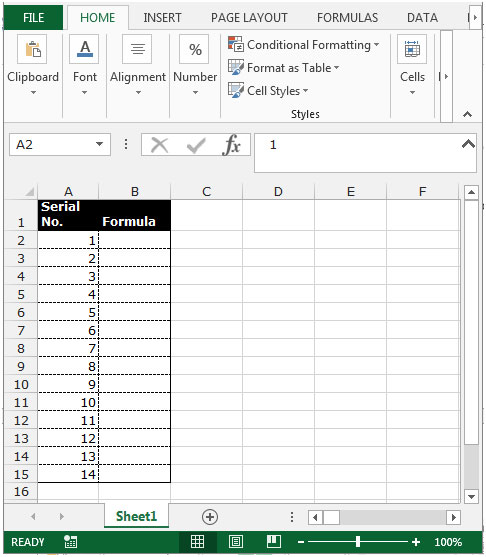Follow the below given steps:-

• Select the cell B2 and write the formula.
• =IF(AND(A2>0,A2<257),IF(A2>26,CHAR(64+INT((A2-1)/26)),"")&CHAR(65+MOD(A2-1,26)),"")
• Press Enter on the keyboard.
• The function will convert the number to the letter.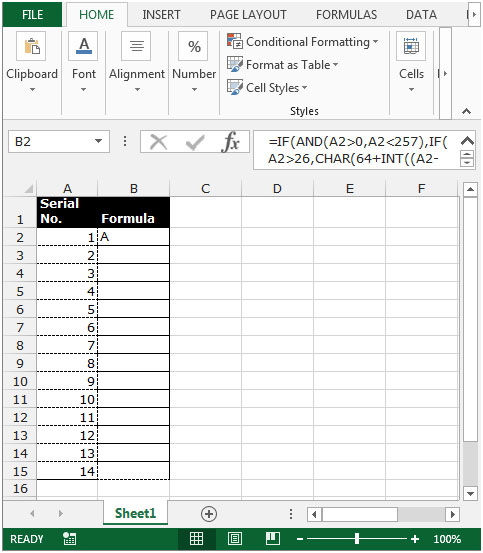• To convert all the serial numbers into letters, copy the same formula by pressing the key Ctrl+Cand paste in the range B3:B15 by pressing the key Ctrl+Von your keyboard.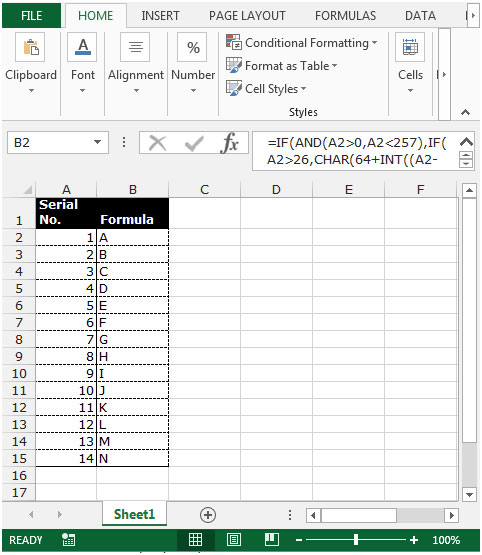Note: This function will convert the serial numbers into letters till number 256. If you want to convert the numbers which are greater than 256, you will need to change the number in the formula.

Terms and Conditions of use

The applications/code on this site are distributed as is and without warranties or liability. In no event shall the owner of the copyrights, or the authors of the applications/code be liable for any loss of profit, any problems or any damage resulting from the use or evaluation of the applications/code.